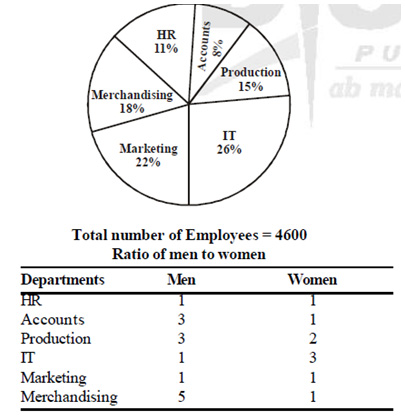# Ace the Quant & DI section of SNAP

Quantitative Aptitude & Data Interpretation plays a crucial role in SNAP test. This section has 20 questions carrying a total of 20 marks. In this article, we will discuss the trends, patterns, commonly asked questions and the strategy to ace the Quantitative Aptitude and Data Interpretation section of the SNAP paper.
###### SNAP - Quantitative Aptitude, Data Interpretation & Data Sufficiency
This section is dominated by arithmetic-based questions. A majority of the questions, which have appeared in this paper over the previous years, have been from topics like percentage, Average, Time, Speed & Distance, Geometry & Mensuration, Profit & Loss etc. There are also some questions on Probability, Logs, Trigonometry, Co-ordinate Geometry and Clocks and Calendars.
The level of difficulty of questions in this section is usually easy to moderate. There are one or two Data Interpretation blocks/sets in this section, and they are of easy to moderate difficulty level. These are usually on pie chart or tables and the related questions are direct and quite manageable with elimination technique. Remember that there is no need for detailed calculations to answer the questions on data interpretation.
Questions on Data Sufficiency have been asked in the SNAP Test, but not on a regular basis, and have been missing in the last few years. However, to be on the safer side, you should cover the basics of this topic.
###### SNAP Previous Year Questions
Here is a quant question from one of the actual SNAP papers:
1. If the numerator of a fraction is increased by 200% and the denominator is increased by 200%, then resultant fraction is 14/5. What is the original fraction?. As you can see, this is a basic percentage-based problem and can be answered without any trouble.
Let us have a look at another quant question in one of the previous years.
2. A can do a piece of work in 25 days and B in 20 days. They work together for 5 days and then A goes away. In how many days will B finish the remaining work?
This is again a very simple question based on time & work topic and anyone who has basic knowledge of the topic can solve it easily.
Similarly, you can have an idea of DI question as follows:
3. Study the pie chart and table carefully to answer the questions below:
Number of employees working in various departments of an organization and the ratio of men to women are given:i) What is the number of women in the accounts department?
ii) What is the total number of employees working in the IT department and HR department together?
iii) What is the ratio of the total number of men to the total number of women working in all the departments together ?
iv) The number of women in the merchandising department forms what per cent of the total number of employees in the organization?
v) What is the ratio of the number of men in the production department to the number of men in the marketing department ?
###### Conclusion
To conclude, since the difficulty level of this section is on an easy to medium side, you should maximize the number of questions attempted to get as much score as possible. If you have brushed up the fundamentals of above-said topics before the test can score very well in this section. Besides this, revision of the shortcuts and calculation techniques will go a long way in helping you solve both DI and Quant problems.